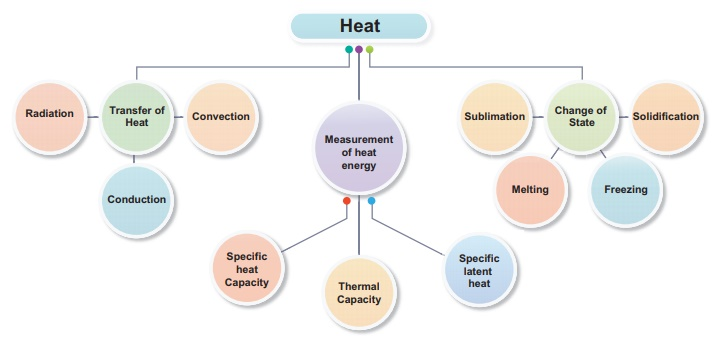Home | | Science 9th std | Introduction

# Introduction

All the substances in our surrounding are made up of molecules. These molecules are generally at motion and posses kinetic energy.

Heat

Introduction

All the substances in our surrounding are made up of molecules. These molecules are generally at motion and posses kinetic energy. At the same time each molecule exerts a force of attraction on other molecules and so they posses potential energy. The sum of the kinetic and potential energy is called the internal energy of the molecules. This internal energy, when flows out, is called heat energy. This energy is more in hot substances and less in cold substances and flows from hot substances to cold substances. In this lesson you will study about how this heat transfer takes place. Also you will study about the effect of heat, heat capacity, change of state and latent heat.Tags : Heat , 9th Science : Heat
Study Material, Lecturing Notes, Assignment, Reference, Wiki description explanation, brief detail
9th Science : Heat : Introduction | Heat